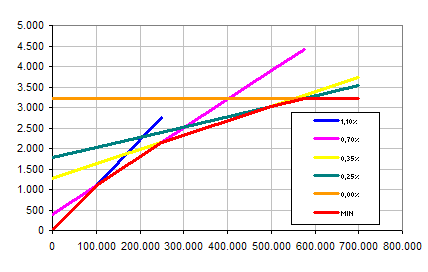@ > Home > Contents > Very short concatenated linear functions via MIN and MAX Excel 4+

Status quo:

Concatenated linear functions by VLOOKUPs are often unnecessarily long, because within f(x) = mx+n you need separate VLOOKUPs for m, x und n. Example:

=(A2%%
-VLOOKUP(A2%%,{0,10,25,50,57.5},1))
*VLOOKUP(A2%%,{0\110,10\70,25\35,50\25,57.5\0},2)
+VLOOKUP(A2%%,{0\0,10\1100,25\2150,50\3025,57.5\3212.5},2)

It calculates fees of 1.1% until 100,000, 0.7% until 250,000, 0.35% until 500,000, 0.25% until 575,000 Euros and none above (A2). %% scales the formula down for shorter constants. Obligatory remark: VLOOKUPs in 99% of all situations are better off with tables referred to, rather than constant arrays.

Improvement:

=MIN(A2%%*{110,70,35,25}+{0,400,1275,1775},3212.5)

What happened? The additional fee per Euro decreases. That means: Until it reaches the maximum amount of 3212.50 (horizontal line), the function only turns right. It consists of 5 linear functions crossing each other once (10 crossings). The lowest value of all (MIN) is the desired function. The only thing you need to find are the f(0)=n, being {0;400;1275;1775} here. On increasing fees per Euro ("left turn"), you would use MAX instead.MIN and MAX do not work within concatenated algebraic functions also using exponential ones. The functions need to be calculated separately as in 5023.htm. Linear step function: 5057.htm.